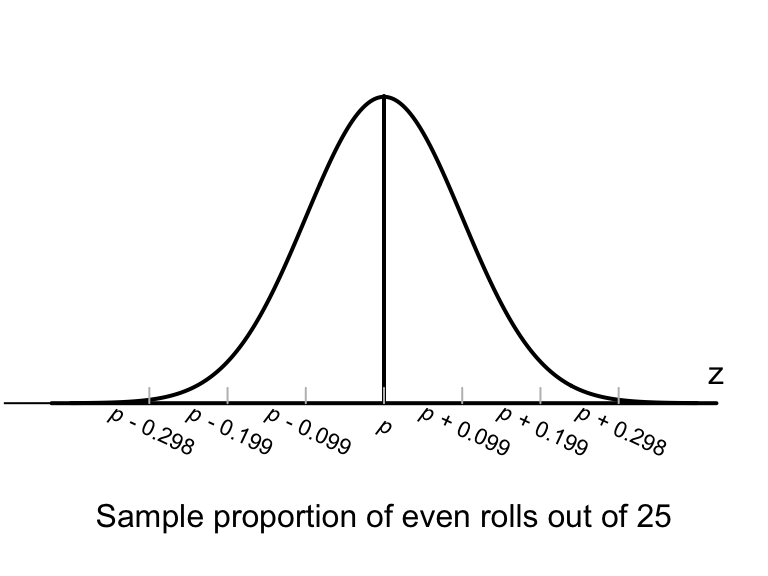## 20.3 Sampling distribution: Unknown proportion

In the die example (Sect. 20.1), an equation was given for computing the standard error for the sample proportion for samples of size $$n$$, when the value of $$p$$ was known.

However, usually the value of $$p$$ (the parameter) is unknown; after all, the reason for taking a sample is to estimate the unknown value of $$p$$. When $$p$$ is unknown, the best available estimate can be used, which is $$\hat{p}$$. When the value of $$p$$ is unknown, the standard error of the sample proportion (written $$\text{s.e.}(\hat{p})$$) is approximately

$\text{s.e.}(\hat{p}) = \sqrt{\frac{ \hat{p} \times (1-\hat{p})}{n}}.$

Definition 20.2 (Sampling distribution of a sample proportion when $$p$$ is unknown) When the value of $$p$$ is unknown, the sampling distribution of the sample proportion is described by

• an approximate normal distribution,
• centred around the (unknown) mean of $${p}$$,
• with a standard deviation (called the standard error of $$\hat{p}$$) of

$\begin{equation} \text{s.e.}(\hat{p}) = \sqrt{\frac{ \hat{p} \times (1-\hat{p})}{n}}, \tag{20.3} \end{equation}$

when certain conditions are met, where $$n$$ is the size of the sample, and $$\hat{p}$$ is the sample proportion.

In general, the approximation gets better as the sample size gets larger.

Let’s pretend for the moment that the proportion of even rolls of a fair die is unknown (to demonstrate some points). In this case, an estimate of the proportion of even rolls can be found by rolling a die $$n=25$$ times and computing $$\hat{p}$$.

Suppose 11 of the $$n=25$$ rolls produced an even number, so that $$\hat{p} = 11/25 = 0.44$$. Then (from Definition 20.2),

$\text{s.e.}(\hat{p}) = \sqrt{ \frac{ 0.44 \times (1 - 0.44)}{25}} = 0.099277.$ (This is very similar to the value of 0.1, the value of the standard error when the value of $$p$$ was known; see Eq. (20.2).)

Hence, the sample proportions would vary with an approximate normal distribution (Fig.20.3), centred around the unknown value of $$p$$ with a standard deviation of $$\text{s.e.}(\hat{p}) = 0.099277$$.FIGURE 20.3: The normal distribution, showing how the proportion of even rolls varies when a die is rolled 25 times

Using the 68–95–99.7 rule again:

About 95% of the values of $$\hat{p}$$ are expected to be between $$p - 0.199$$ and $$p + 0.199$$.

Though we are pretending the value of $$p$$ is unknown, the value of $$\hat{p}$$ is known however. What if the roles of $$p$$ and $$\hat{p}$$ were ‘reversed?’ Then,

About 95% of the values of $$p$$ are expected to be between $$\hat{p} - 0.199$$ and $$\hat{p} + 0.199$$.

Since $$\hat{p} = 0.44$$, this is equivalent to:

About 95% of the values of $$p$$ are expected to be between $$0.24$$ and $$0.64$$.

This interpretation is not quite correct, but the idea seems reasonable. This is called a confidence interval (or CI), based on ideas from Sect. 20.2.

In summary, using $$\hat{p} = 0.44$$ and $$\text{s.e.}(\hat{p}) = 0.0993$$, the (approximate) 95% CI is

$0.44 \pm (2 \times 0.0993),$ or from 0.241 to 0.639. This CI straddles the population proportion of $$p=0.5$$, though we would not know this if $$p$$ truly was unknown.

In this case, we know the value of the population parameter: $$p = 0.5$$.

Usually we do not know the value of the parameter: that’s why we are taking a sample.